# How to write a Transformer Recipe for Driverless AIBy Ashrith Barthur | minute read | November 18, 2019## What is a transformer recipe?

A transformer (or feature) recipe is a collection of programmatic steps, the same steps that a data scientist would write a code to build a column transformation. The recipe makes it possible to engineer the transformer in training and in production. The transformer recipe, and recipes, in general, provide a data scientist the power to enhance the strengths of H2O DriverlessAI with custom recipes. These custom recipes would bring in nuanced knowledge about certain domains – i.e. financial crimes, cybersecurity, anomaly detection. etc. It also provides the ability to extend DriverlessAI to solve custom solutions for time-series.

## How to write a simple DAI recipe?

The structure of a recipe that works with DriverlessAI is quite straight forward.

1. DriverlessAI provides a `CustomTransformer` Base class that needs to be extended for one to write a recipe. The `CustomTransformer` class provides one the ability to add a customized transformation function. In the following example, we are going to create a transformer that will transform a column with the `log10` of the same column. The new column, which is transformed by `log10` will be returned to DriverlessAI as a new column that will be used for modeling.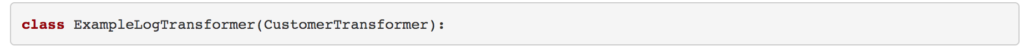The `ExampleLogTransformer` is the class name of the transformer that is being newly created. And in the parenthesis the `CustomTransformer` is being extended.

1. In the next step, one needs to populate the type of problem the custom transformer is solving:
1. Are you solving a regression problem?
2. Are you solving a classification problem that is binary?
3. Are you solving a classification problem that is multiclass?

Depending on what kind of outcome the custom transformer is solving, each one of the above needs to be enabled or disabled. And the following example will show you how this can be done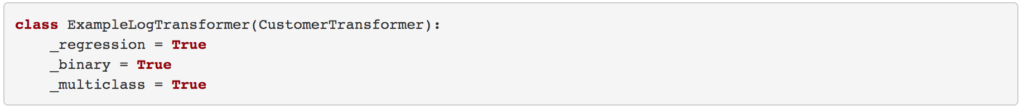In the above example, we are building a `log10` transformer, and this transformer is an application, for a regression, binary, or a multiclass problem. Therefore we set all of those as `True`.

1. In the next step, we tackle four more settings of a transformer. They are as follows:
1. Output Type – What is the output type of this transformer?
2. Reproducibility – Is this a reproducible transformer? Meaning is this transformer deterministic, and deterministic if you can set the seed?
3. Model inclusion/exclusion – Here we describe the type of modeling that uniquely fits, or does not fit the transformer, respectively.
1. Custom package requirements – Does this transformer require any custom packages.In this example, we enable the acceptance test by returning `True` for the `do_acceptance_test` function

1. In the following example, we set the parameters for the type of column that we require as input, the minimum and the maximum number of columns that we need to be able to provide an output, along with the relative importance of the transformer.

The column type or `col_type` can take nine different column data types, and they are as follows: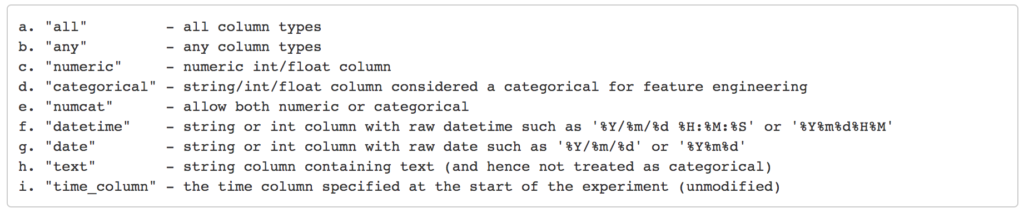Please note that if `col_type` is set to `col_type=all` then all the columns in the dataframe are provided to this transformer, no selection of columns will occur.

The `min_cols` and `max_cols` either take numbers/integers or take string parameters as `all` and `any`. The `all` and `any` should coincide with the same `col_type`, respectively.

The `relative_importance` takes a positive value. If this value is more than `1` then the transformer is likely to be used more often than other transformers in the specific experiment. If it is less than `1` then it is less likely to be used than other transformers in the specific experiment. If it is set to `1` then it is equally likely to be used as other transformers in the specific experiment, provided other transformers are also set to relative importance `1`. i , which will over, or under-representation. Default value is `1`, value greater than `1` is over-representation and under `1` is under-representation.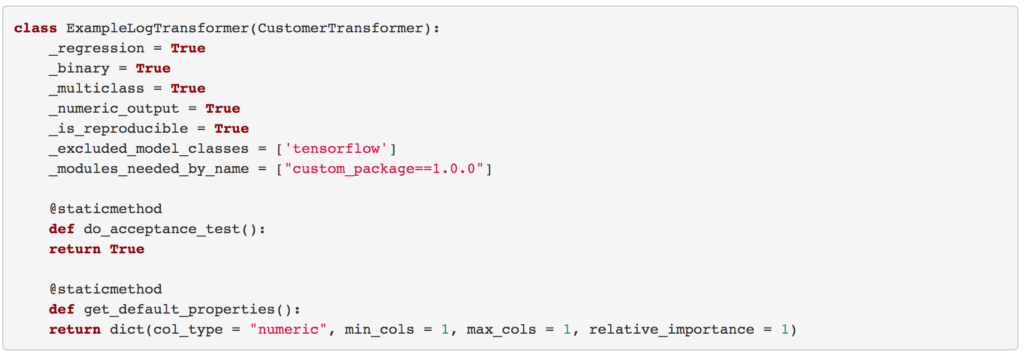In the above example, as we are dealing with a numeric column (recall, that we are calculating the log10 of a given column) we set the `col_type` to `numeric`. We set the `min_cols` and `max_cols` to `1` as we need only one column and the `relative_importance` to `1`.

1. The custom transformer function has two fundamental functions that are required to make a transformer. They are:
1. `fit_transform` This function is used to fit the transformation on the training dataset, and returns the output column.
2. `transform` This function is used to transform the testing or production dataset, and is always applied after the `fit_transform.`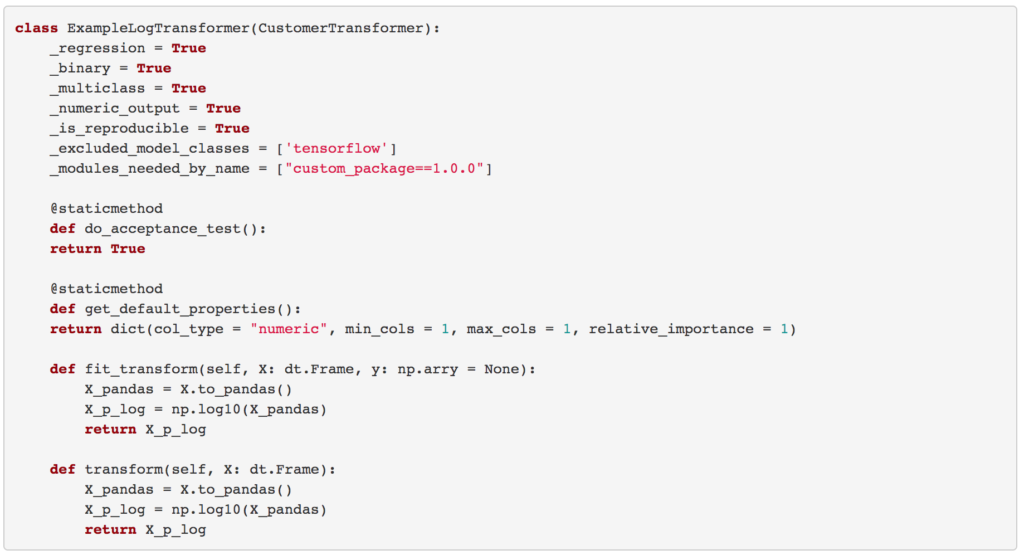In the above example, we compose the `fit_transform` and `transform` for training and testing data, respectively. In the `fit_transform` the response variable `y` is available. Here our dataframe is named `X`. Now `X` will be transformed to pandas frame by using the `to_pandas()` function. Further, a `log10` of the column will be applied and returned. The `to_pandas()` function is described here for ease of understanding. A real-world implementation of log transformer is available at the following link HyperLink to LogTransformer

1. This code is to be stored as a python code file – `example_transform.py`
2. To ingest this code, one needs to first need to add dataset to be modeled upon into DriverlessAI.
3. After ingestion, `predict` is chosen by right-clicking. Following this, a `target` or `response` variable is set.
4. Next, the `Expert Settings` is chosen, following the recipes, and this – `example_transform.py` is ingested.
5. Next, the transform is chosen under Recipes option and the experiment is started.

Want to give it a try? Check out a free demo with the tutorials.